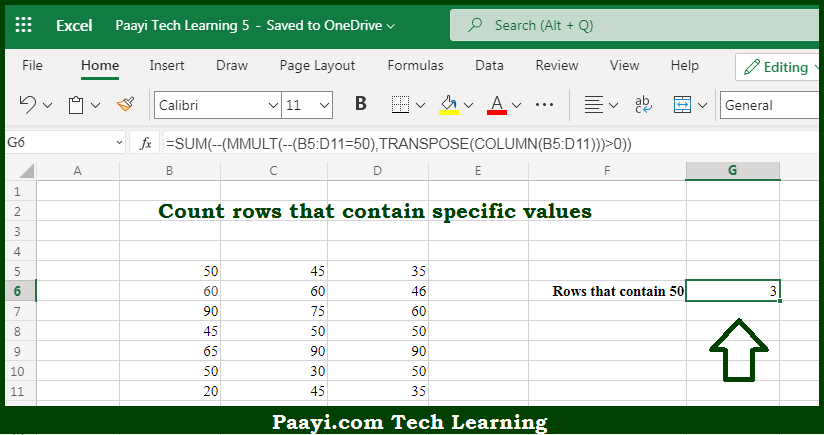# Learn How to Count Rows that Contain Specific Values in Microsoft Excel

Written by | 0 Comments | 534 Views

In this article, you will learn how to COUNT various things in Microsoft Excel using a single or combination of functions and its purpose in Microsoft Excel. You will also get to know how to Count Rows that Contain Specific Values & generic formula

Count Rows that Contain Specific Values in Microsoft Excel

The main purpose of this formula is to count rows that contain specific values. Here we will learn how to count the rows in a workbook containing the specific value in Microsoft Excel. That implies, with the help of a formula based on the array and using functions like   MMULT, TRANSPOSE, COLUMN, and SUM you can able to count rows that contain specific values. So, with the help of this formula, you can able to count paired items in the listed combinations.

General Formula to Count Rows that Contain Specific Values

=SUM(--(MMULT(--(criteria),TRANSPOSE(COLUMN(data)))>0))

The Explanation for the Count Rows that Contain Specific ValuesSo we know that with the help of the given formula above you can able to count rows that contain specific values. Here we will learn how to count the rows in a workbook containing the specific value in Microsoft Excel. As we know that the goal in the example is to count rows containing the specific value. But the tough task is that the value might appear in any of several columns, and might appear in more than one column of the same row. When we start work from the inside out, the logical criteria --(data=90) is used. So, with the help of this formula, you can able to count rows that contain specific values.